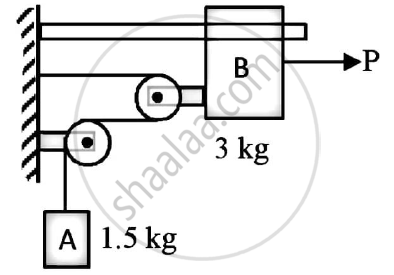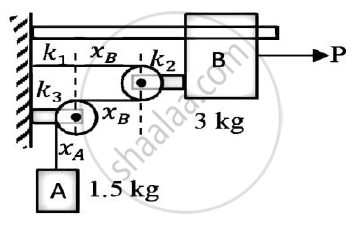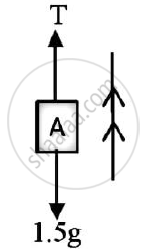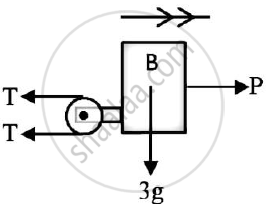# The System in Figure is Initially at Rest. Neglecting Friction Determine the Force P Required If the Velocity of the Collar is 5 M/S After 2 Sec and Corresponding Tension in the Cable. - Engineering Mechanics

The system in figure is initially at rest.
Neglecting friction determine the force P required if the velocity of the collar is 5 m/s after 2 sec and corresponding tension in the cable.#### Solution

Solution :
For block B
u = 0
t = 2 s
v = 5m/s
a=(5-0)/2=2.5m//s^2…………..(1)
Assume the string across the two pulleys be of length L
Assume xA and xB be the displacements of block A and collar B respectively
Assume k1,k2 and k3 be the lengths of the string which remain constant irrespective of the position of block A and block Bk1 + xB + k2 + xB + k3 + xA = L
xA = L - k1 - k2 - k3 - 2xB
Differentiating with respect to time
vA = -2vB
Differentiating with respect to time one again
aA = -2aB
Considering only magnitude
aA = 2aB
aA = 2 x 2.5
= 5 m/s2 ………..(2) (From 1)
Weight of block A(WA) = mAg
= 14.715 N
Assume T to be the tension in the string
Consider the vertical motion of block A
F.B.D of block AΣFy = mAaA
T – WA = mAaA
T - 14.715 = 1.5 x 5
T - 14.715 = 1.5 x 5  …………..(3)
Consider the horizontal motion of collar B
F.B.D of collar BΣFx = mBaB
P - 2T = mBaB
P - 2x22.215 = 3x2.5
P = 51.93 N
Force P required = 51.93 N
Tension in the cable = 22.215 N

Concept: Introduction to Laws of Friction
Is there an error in this question or solution?